• +91 9971497814
• info@interviewmaterial.com

# Chapter 11- Thermal Properties of matter Interview Questions Answers

### Related Subjects

Question 1 : The triple points of neon and carbon dioxide are 24.57 K and 216.55 Krespectively. Express these temperatures on the Celsius and Fahrenheit scales.

Answer 1 : The relation between kelvin scale and Celsius scale is T_K – 273.15 =T_C => T_C=T_K– 273.15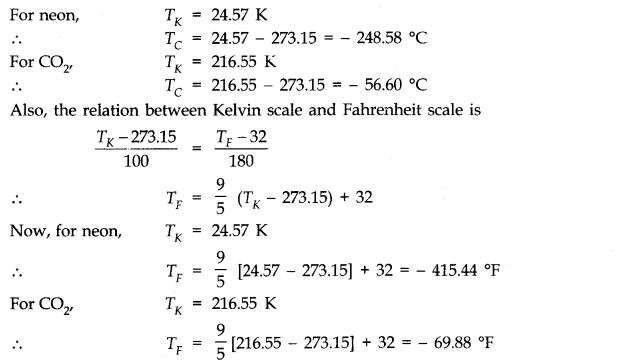Question 2 : Two absolute scales A and B have triple points of water defined to be 200 A and 350 B. What is the relation between TA and TB ?

Answer 2 : As we know, triple point of water on absolute scale = 273.16 K, Size of one degree of kelvin scale on absolute scale A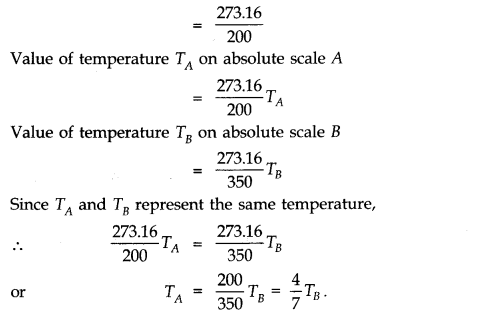Question 3 : The-electrical resistance in ohms of a certain thermometer varies with temperature according to the approximate law: R = R0 [1+ α (T – T0)].
The resistances is 101.6 Ωat the triple-point of water 273.16 K, and 165.5 Ωat the normal melting point of lead (600.5 K).What is the temperature when the resistance is 123.4 Ω ?

Answer 3 : Here, R0 = 101.6 Ω; T0 = 273.16 K Case(i) R1= 165.5 Ω; T= 600.5 K, Case (ii) R2 =123.4 , T2 = ?
Using the relation R = R0[1 + α (T – T0)]
Case (i) 165.5 = 101.6 [1 + α (600.5 – 273.16)]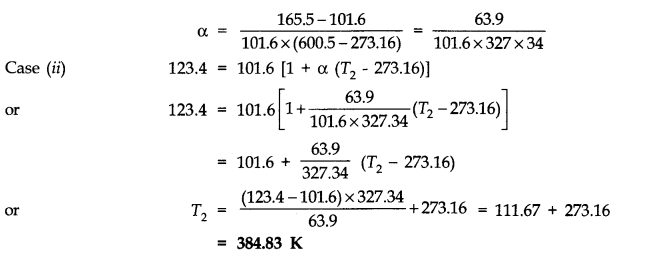Question 4 : Answer the following:
(a) The triple-point of water is astandard fixed point in modem thermometry. Why ? What is wrong in taking themelting point of ice and the boiling point of water as standard fixed points(as was originally done in the Celsius scale) ?
(b) There were two fixed points in the original Celsius scale as mentioned above which were assigned the number 0 °C and 100 °C respectively. On the absolute scale, one of the fixed points is the triple-point of water, which on the Kelvin absolute scale is assigned the number 273.16K. What is the other fixed point on this (Kelvin) Scale ?
(c) The absolute temperature (Kelvin scale) T is related to the temperature tc on the Celsiusscale tc = T – 273.15
Why do we have 273.15 in this relation, and not 273.16 ?
(d) What is the temperature of the triple-point of water on an absolute scale whose unit interval size is equal to that of the Fahrenheit scale ?

Answer 4 : (a) Triple point of water has a unique value i.e., 273.16 K. The melting point and boiling points of ice and water respectively do not have unique values and change with the change in pressure.
(b) On Kelvin’s absolute scale, there is only one fixed point, namely, the triple-point of water and there is no other fixed point.
(c) On Celsius scale 0 °C corresponds to the melting point of ice at normal pressure and the value of absolute temperature is 273.15 K. The temperature273.16 K corresponds to the triple point of water.
(d)The Fahrenheit scale and Absolute scale are related as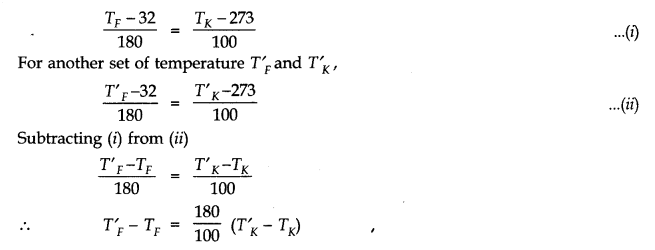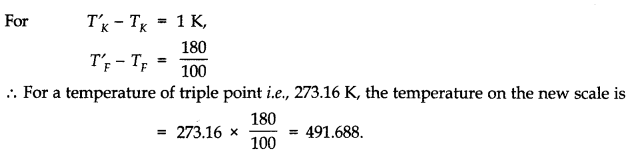Question 5 : Two ideal gas thermometers A and B use oxygen and hydrogen respectively. The following observations are made: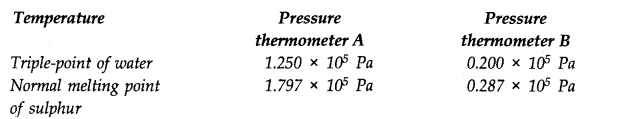(a) What is the absolute temperature of normal melting point of sulphur as read by thermometers A and B ?
(b) What do you think is the reason behind the slight difference in answers of thermometers A and B ? (The thermometers are not faulty). What further procedure is needed in the experiment to reduce the discrepancy between the two readings ?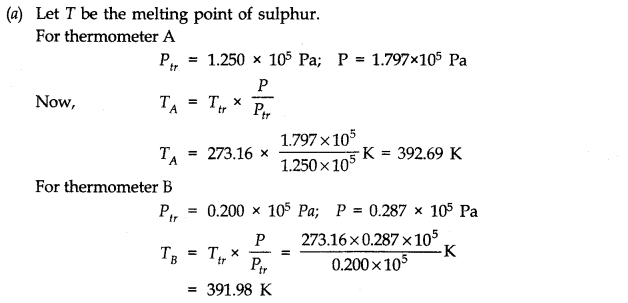(b) The value of the melting point of sulphur found from the two thermometers differ slightly due to the reason that in practice, the gases do not behave strictly as perfect gases i.e., gases are not perfectly ideal.
To reduce the discrepency, readings should be taken for lower and lower pressures and the plot between temperature measured versus absolute pressure of the gas at triple point should be extrapolated to obtain the temperature in the limit pressure tends to zero (if P —> 0), when the gases approach ideal gas behaviour.

Question 6 : A steel tape 1m long is correctly calibrated for a temperature of 27.0 °C. The length of a steel rod measured by this tape is found to be 63.0 cm on a hot day when the temperature is 45.0 °C. What is the actual length of the steel rod on that day? What is the length of the same steel rod on a day when the temperature is 27.0 °C? Coefficient of linear expansion of steel = 1.20 × 10–5 K–1

T = 27 °C

At the temperature of 27 °C the length of the tape is 1m =100 cm

T1 = 45°C

At the temperature of 45 °C the length of the tape is 63 cm

Coefficient of linear expansion of steel = 1.20 × 10–5 K–1

Let L be the actual length of the steel rod and L’ is the lengthat 45 °C

L’ = L [1 + (T1 – T)]

= 100 [1+ 1.20 × 10–5 (45-27)]

= 100[1+ 21.6 × 10–5]

= 100 + 2160 x 10–5

= 100 + 0.02160 = 100.02160

The actual length of the rod at 450 Cis

L2 =(100.02160 /100) x 63 = 63. 013608 cm

The length of the rod at 270 Cis 63.0 cm

Question 7 : A large steel wheel is to befitted on to as haft of the same material. At 27 °C, the outer diameter of the shaft is 8.70cm and the diameter of the central hole in the wheel is, 8.69 cm. The shaft is cooled using ‘dry ice’. At what temperature of the shaft does the wheel slip on the shaft ? Assume coefficient of linear expansion of the steel to be constant over the required temperature range αsteel= 1-20 x 10-5K-1.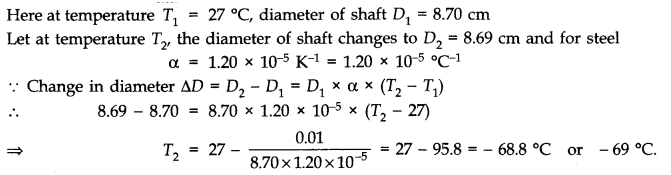Question 8 : A hole is drilled in a copper sheet. The diameter of the hole is 4.24 cm at 27.0 °C. What is the change in the diameter of the hole when the sheet is heated to 227 °C ? Coefficient of linear expansion of copper = 1.70 x 10-5K-1.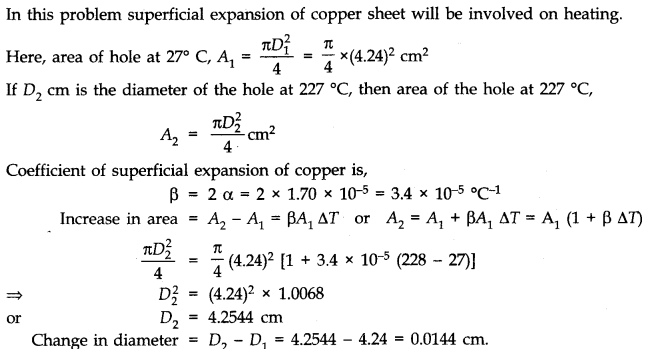Question 9 : A brass wire 1.8 m long at 27 °C is held taut with little tension between two rigid supports. If the wire is cooled to a temperature of – 39 °C, what is the tension developed in the wire, if its diameter is 2.0 mm ? Co-efficient of linear expansion of brass = 2.0 x 10-5K-1; Young’s modulus of brass = 0.91 x 1011 Pa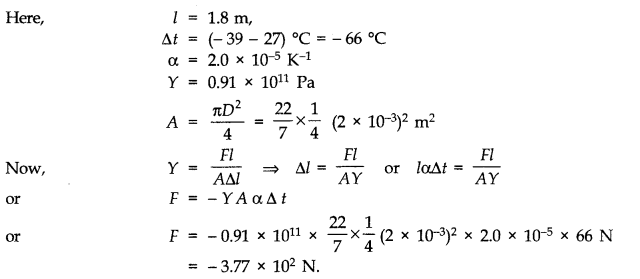Question 10 : A brass rod of length 50 cm and diameter 3.0 mm is joined to a steel rod of the same length and diameter. What is the change in length of the combined rod at 250 °C, if the original lengths are at 40.0 °C ? Is there a ‘thermal stress’ developed at the junction ? The ends of the rod are free to expand (Co-efficient of linear expansion of brass = 2.0 x 10-5 °C-1, steel = 1.2 x 10-5 °C-3.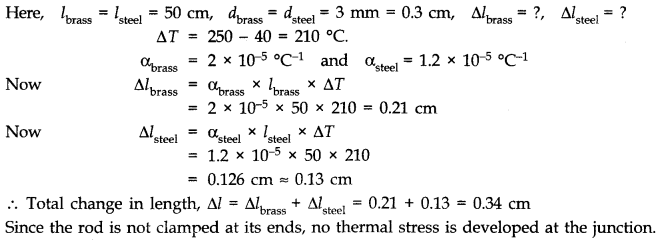Todays Deals### Chapter 11- Thermal Properties of matter Contributorskrishan

Name:
Email:

# Latest News# 9000 interview questions in different categories